# 几款连衣裙的设计和纸样

11687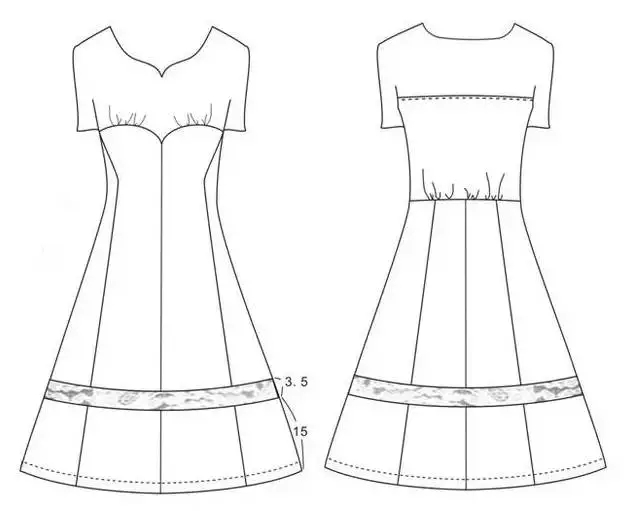1、部位尺寸（单位：cm）

2、测量方法：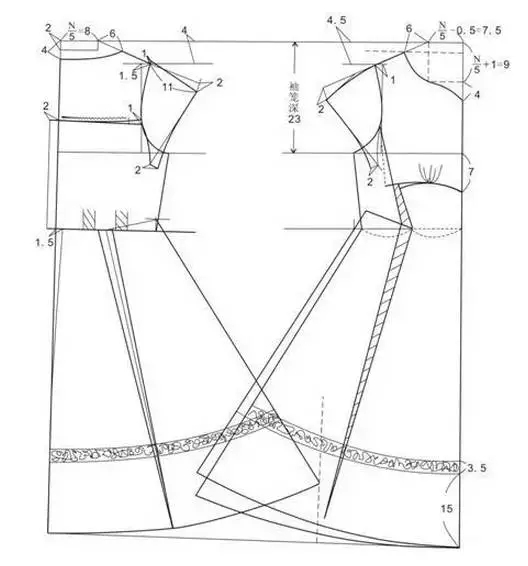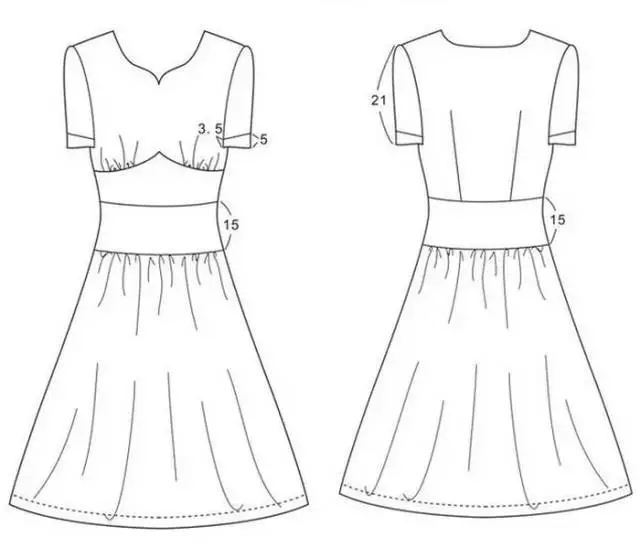1、部位尺寸（单位：cm）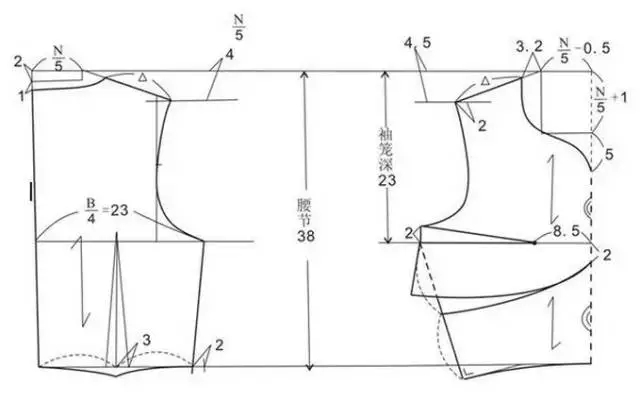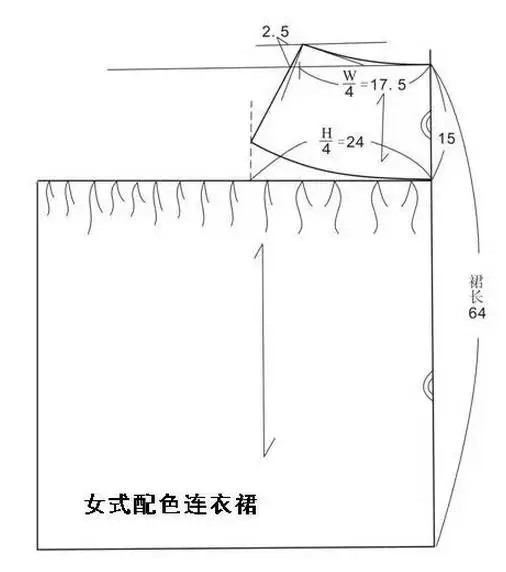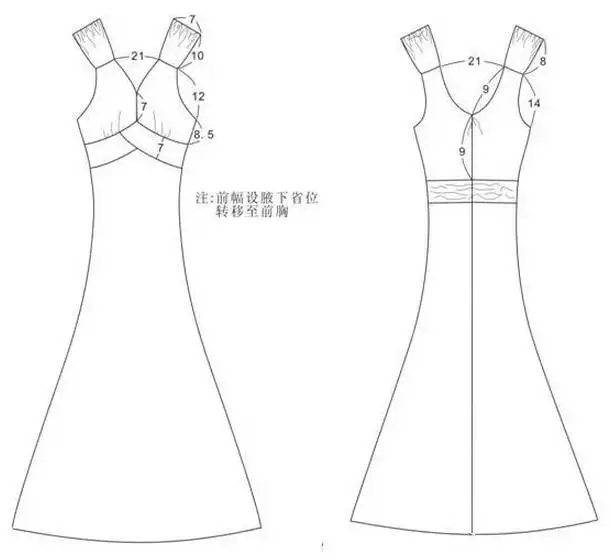1、部位尺寸（单位：cm）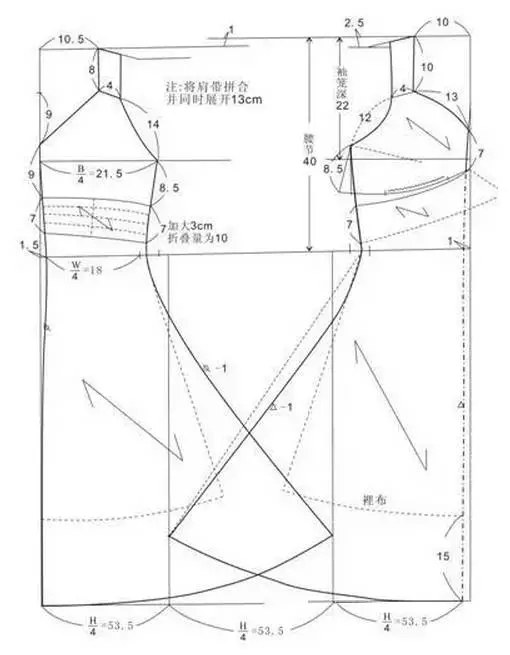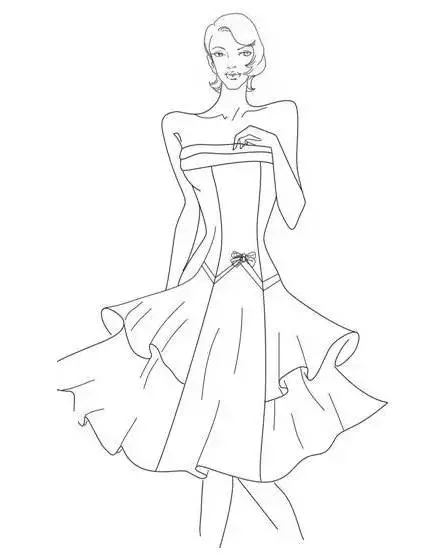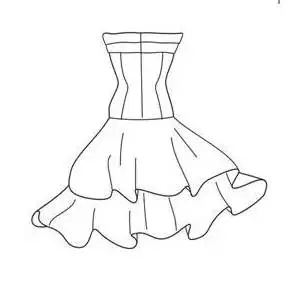1、款式特点

2、部位尺寸（单位：cm）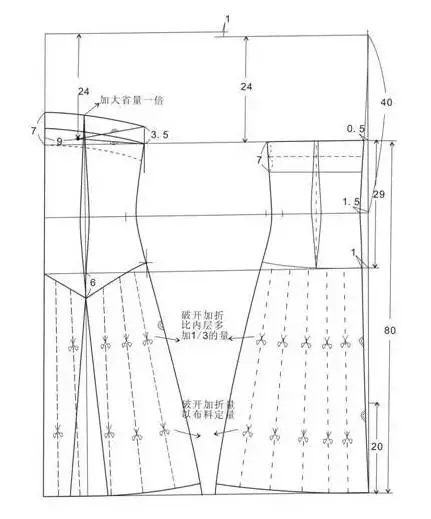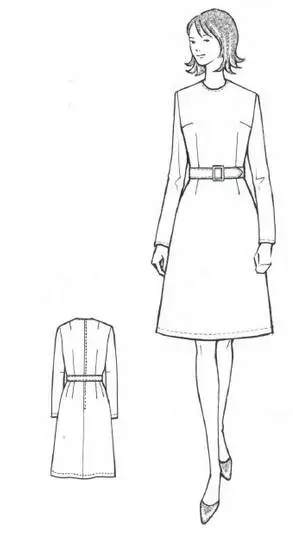1、款式特点

2、部位尺寸（单位：cm）

L=0.6h+4=100cm

WL=0.25h=40cm

SL=0.3h+8=56cm

BL=0.2B+3+2=24cm

B=B*+10cm=94cm

B-W=17cm，臀长=0.1h+2=18cm

H=B=90+4=94cm

N=0.2B*+20≈39cm

S=0.25B+（15-16）≈39cm

3、衣身结构平衡

4、制图方法

①作基本衣身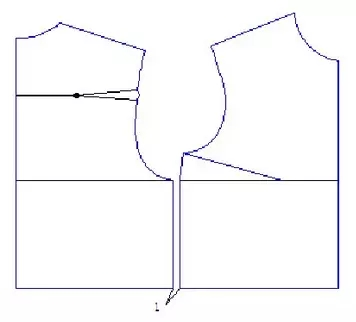②卡腰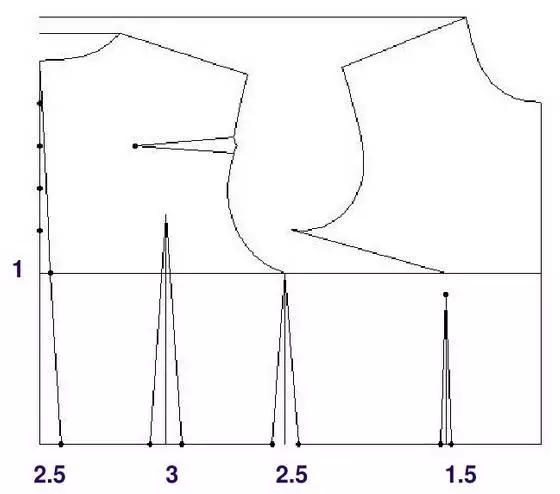③省道转移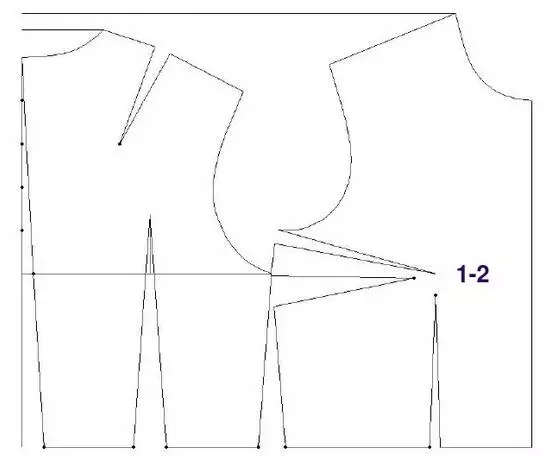④省道转移至腰省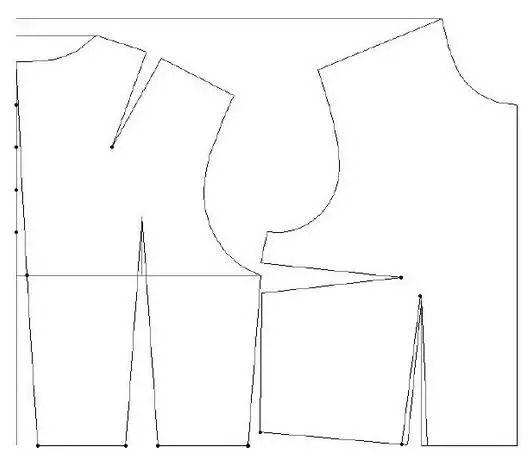⑤补足胸围，调整腰节长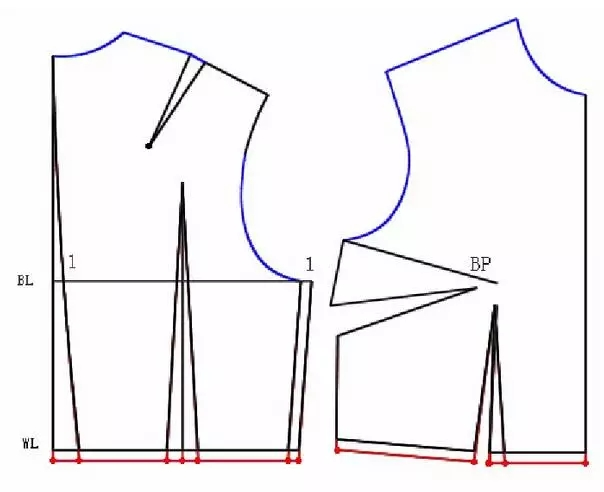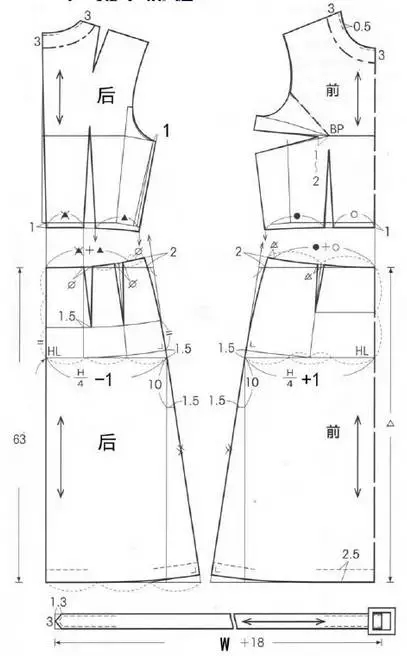5